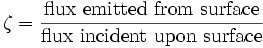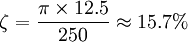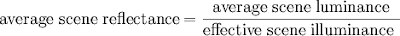## Wednesday, June 18, 2008

### Calibrated Reflectance

It is commonly stated that reflected-light meters are calibrated to an 18% reflectance, but the calibration has nothing to do with reflectance, as should be evident from the exposure formulas. However, some notion of reflectance is implied by a comparison of incident- and reflected-light meter calibration.

Combining the reflected-light and incident-light exposure equations and rearranging givesReflectance ζ is defined asA uniform perfect diffuser (i.e., one following Lambert's cosine law) of luminance L emits a flux density of πL; reflectance then isIlluminance is measured with a flat receptor. It is straightforward to compare an incident-light measurement using a flat receptor with a reflected-light measurement of a uniformly illuminated flat surface of constant reflectance. Using values of 12.5 for K and 250 for C givesWith a K of 14, the reflectance would be 17.6%, close to that of a standard 18% neutral test card. In theory, an incident-light measurement should agree with a reflected-light measurement of a test card of suitable reflectance that is perpendicular to the direction to the meter. However, a test card seldom is a uniform diffuser, so incident- and reflected-light measurements might differ slightly.

In a typical scene, many elements are not flat and are at various orientations to the camera, so that for practical photography, a hemispherical receptor usually has proven more effective for determining exposure. Using values of 12.5 for K and 330 for C givesWith a slightly revised definition of reflectance, this result can be taken as indicating that the average scene reflectance is approximately 12%. A typical scene includes shaded areas as well as areas that receive direct illumination, and a wide-angle averaging reflected-light meter responds to these differences in illumination as well as differing reflectances of various scene elements. Average scene reflectance then would bewhere “effective scene illuminance” is that measured by a meter with a hemispherical receptor.

ISO 2720:1974 calls for reflected-light calibration to be measured by aiming the receptor at a transilluminated diffuse surface, and for incident-light calibration to be measured by aiming the receptor at a point source in a darkened room.

For a perfectly diffusing test card and perfectly diffusing flat receptor, the comparison between a reflected-light measurement and an incident-light measurement is valid for any position of the light source. However, the response of a hemispherical receptor to an off-axis light source is approximately that of a cardioid rather than a cosine, so the 12% “reflectance” determined for an incident-light meter with a hemispherical receptor is valid only when the light source is on the receptor axis.